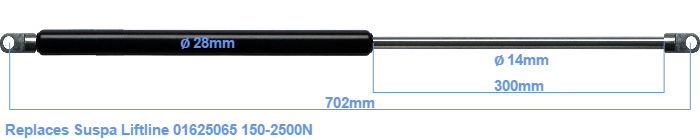# Replacement for Suspa Liftline 01625065 150-2500N

\$52.20

Replacement gas spring for the Suspa Liftline 01625065 150-2500 Newton. The eye has a thickness of 10mm and a hole diameter of 10.1mm. Brand: Stabilus Industry Line.

Please note:
The thickness of the eye of this replacement is 10mm instead of 12mm.
 Force Choose an option150 Newton200 Newton250 Newton300 Newton350 Newton400 Newton450 Newton500 Newton550 Newton600 Newton650 Newton700 Newton750 Newton800 Newton850 Newton900 Newton950 Newton1000 Newton1050 Newton1100 Newton1150 Newton1200 Newton1250 Newton1300 Newton1350 Newton1400 Newton1450 Newton1500 Newton1550 Newton1600 Newton1650 Newton1700 Newton1750 Newton1800 Newton1850 Newton1900 Newton1950 Newton2000 Newton2050 Newton2100 Newton2150 Newton2200 Newton2250 Newton2300 Newton2350 Newton2400 Newton2450 Newton2500 NewtonClear
This gas spring is also known as 16-6 016 25065, 16-6-368-300-A31-B31.The diameter of the cylinder of this gas spring is 28 mm. The rod has a diameter of 14 mm. The rod (the chrome part) is 300 mm long. In total, the length is 702 millimeter. Keep in mind that this is the length between the centers of the mounting parts. The distance from thread to thread (without mounting parts) is 648 mm. This replacement gas spring (01625065) has a force of 150-2500 Newton (N). This is not an original gas spring produced by Suspa Liftline, but it can replace a Suspa Liftline gas spring. HAHN Gasfedern, a Stabilus subsidiary, is responsible for the production of this replacement gas spring. The gas spring has the brand Stabilus Industry Line. The force (150-2500 N) and dimensions are almost entirely identical to the original.
Category: